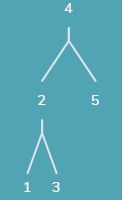• ## 利用层次遍历非递归求二叉树高度

万次阅读 多人点赞 2016-09-30 17:47:42
利用层次遍历非递归求二叉树高度主要的思想是：一层一层地出队列 — 在我们每次访问完一层时，这时队列中存储的刚好是下一层的所有元素。所以在下一次循环开始时，首先记录该层的元素个数，一次性访问完这一层的所有...
leetcode 104. Maximum Depth of Binary Tree
求二叉树的最大深度，也即其高度。
递归版本比较容易理解。利用层次遍历非递归求二叉树高度主要的思想是：一层一层地出队列 — 在我们每次访问完毕一层时，这时队列中存储的刚好是下一层的所有元素。所以在下一次循环开始时，首先记录该层的元素个数，一次性访问完这一层的所有元素。
/**
* Definition for a binary tree node.
* struct TreeNode {
*     int val;
*     TreeNode *left;
*     TreeNode *right;
*     TreeNode(int x) : val(x), left(NULL), right(NULL) {}
* };
*/
class Solution {
public:
int maxDepth(TreeNode* root) {
//return maxDepthWithLevelTraversal(root);
return maxDepthRecursion(root);
}

/* 递归版本 */
int maxDepthRecursion(TreeNode* root){
int HL, HR, MaxH;
if (root){
HL = maxDepth(root->left);
HR = maxDepth(root->right);
MaxH = (HL > HR) ? HL : HR;
return MaxH + 1;
}
else
return 0;
}

/* 层次遍历的非递归版本 */
int maxDepthWithLevelTraversal(TreeNode* root){
/* 判断树是否为空 */
if(!root){
return 0;
}

int height = 0;    // 初始化树的高度为0

queue<TreeNode*>Q;  // 初始化一个队列，并将根节点入队
Q.push(root);

/* 当队列不为空时 */
/* 实际上当每次循环开始时，队列中存储的刚好是将要访问下一层的所有元素*/
while(!Q.empty()){
height++;
int curLevelSize = Q.size();  // 记录当前层元素个数
int cnt = 0;
/* 弹出当前层所有元素 */
while(cnt < curLevelSize){
TreeNode* temp = Q.front();
Q.pop();
cnt++;
/* 将下一层的元素入队列 */
if(temp->left){
Q.push(temp->left);
}
if(temp->right){
Q.push(temp->right);
}
}
}
return height;
}
};
继续思考上面的非递归代码还用来做什么？比如求树的宽度，也就是元素个数最多的那一层元素个数。记录所有层间的最大值即可。
leetcode 111. Minimum Depth of Binary Tree  这题是求二叉树的最小高度，与最大高度类似，不同之处在于层次遍历时，如果在该层遇到有叶子节点则立即返回，在进行层次遍历时需要进行叶子节点的判断。
/**
* Definition for a binary tree node.
* struct TreeNode {
*     int val;
*     TreeNode *left;
*     TreeNode *right;
*     TreeNode(int x) : val(x), left(NULL), right(NULL) {}
* };
*/
class Solution {
public:
int minDepth(TreeNode* root) {
if(!root)
return 0;

queue<TreeNode*>Q;
Q.push(root);

int height = 0;

while(!Q.empty()){
height++;

int curLevelSize = Q.size();
int cnt = 0;

while(cnt++ < curLevelSize){
TreeNode *temp = Q.front();
Q.pop();
/* 此处比二叉树高度多了叶子节点的判断 */
if(!temp->left && !temp->right)
return height;

if(temp->left)
Q.push(temp->left);
if(temp->right)
Q.push(temp->right);
}
}
return height;
}
};
展开全文层次遍历 数据结构
• 题目：假设二叉树采用二叉链表存储结构，设计一个非递归算法求二叉树高度 分析： 若要采用非递归的方式来求得二叉树高度，我们采用层次遍历是最合适的，因为这一层一层的不就很好数吗哈哈。具体实现： 这里...
     题目：假设二叉树采用二叉链表存储结构，设计一个非递归算法求二叉树的高度     分析：         若要采用非递归的方式来求得二叉树的高度，我们采用层次遍历是最合适的，因为这一层一层的不就很好数吗哈哈。具体实现：         这里唯一的难点就在于我们如何得知高度该加一了；我们可以设置一个标志num用来记录每一层入栈的节点个数，当我们出栈数         达到该数值时也就意味着我们的高度该加一了。
代码
struct biTree {
char data;
struct biTree *lchild;
struct biTree *rchild;
};
struct Squeue {
biTree *arr;
int front, rear;
};

#include <stdio.h>
#include <stdlib.h>

int getHigh(biTree *T,Squeue *sq,int maxSize) {
int oldNum=0,curNum=0,high=0;//记录一层有多少节点
struct biTree *p = T;
struct biTree *r=(struct biTree *)malloc(sizeof(struct biTree));
bool enQueueS(Squeue *, biTree *, int );
bool isEmpty(Squeue *);
bool deQueueS(Squeue *, biTree *, int);

enQueueS(sq,p,maxSize);//将根节点入队
oldNum++;//此时队列中只有一个节点

while (!isEmpty(sq)) {
deQueueS(sq,r,maxSize);//取出队首元素
if (r->lchild) {
curNum++;//下一层的节点数+1

展开全文队列 数据结构 算法
• int Hight(Node* root) { if ( !root ) return 0; else return max(Hight(root->left_child),Hight (root->right_child)) + 1 }数据结构与算法
• 此问题可拆解为左右子树最高的高度加1，递归出口为访问到叶子节点本身时其高度为1，此时返回0即可。 class Solution{ public int height(TreeNode root){ if(root == null){ return 0; } return Math.max...
此问题可拆解为求左右子树最高的高度加1，递归出口为访问到叶子节点本身时其高度为1，此时返回0即可。
class Solution{
public int height(TreeNode root){
if(root == null){
return 0;
}
return Math.max(height(root.left),height(root.right)) + 1;
}
}由于使用了递归，内存开销巨大，不过不失为一种简单的方法求树高。
展开全文• 假设二叉树采用二叉链表存储结构，设计一个非递归算法求二叉树高度。 参数： 辅助队 q（存储节点）；1对位标记参数L（用来记录当前一层队尾所在位置）；指针P（用来指向出队元素）；记录高度参数 dept（记录当前高度...
题目：
假设二叉树采用二叉链表存储结构，设计一个非递归算法求二叉树高度。
参数：
辅助队 q（存储节点）；1对位标记参数L（用来记录当前一层队尾所在位置）；指针P（用来指向出队元素）；记录高度参数 dept（记录当前高度）；
步骤：
参数初始化 L=0，deot=0；将结点放入队列q中，判断当前结点是否有孩子结点，有则将孩子结点放入队列q中判断L是否等于队头指针，若等于则dept+1判断队头指针是否小于队尾指针，若小于则继续进行循环，若不小于则循环结束返回当前dept值；
代码实现：
#include <iostream>
using namespace std;

typedef struct TreeNode{
char data;
struct TreeNode *lchrld,*rchild;
int tag;
}TreeNode,*Tree;

//构建树
void creatTree(Tree &t){
char ch;
ch = getchar();
if(ch=='#') t=NULL;
else{
t = (TreeNode *)malloc(sizeof(TreeNode));
t->data = ch;
t->tag=0;
t->lchrld=NULL;
t->rchild = NULL;
creatTree(t->lchrld);
creatTree(t->rchild);
}

}
int dept(Tree t){

if(!t) return 0;
TreeNode *q;
int f=-1,r=-1;
int ans=0,L=0;
TreeNode *p;
q[++r] = t;
while (f<r)
{
p=q[++f];
if (p->lchrld) q[++r] = p->lchrld;
if(p->rchild) q[++r] = p->rchild;
if(L==f){
ans++;
L=r;
}

}
return ans;

}
int main(){
Tree t;
creatTree(t);
cout<<dept(t);

return 0;
}


测试用例：输入样式 421##3##5##展开全文算法 数据结构
• 以下是打印二叉树树形结构的要求： 第二部分为表达式树的显示，如样例输出所示。如果该二叉树是一棵满二叉树，则最底部的叶子结点，分别占据横坐标的第1、3、5、7……个位置（最左边的坐标是1），然后它们的父结点...树形结构
• 递归计算二叉树高度Previously I wrote about an algorithm for finding out the height of a binary tree using iteration. Though that method gets the job done (in 7 steps no less), the same can be ...python java 算法 编程语言
• 开始接触二叉树，记录自己对递归求解二叉树的理解。 代码地址 def depth_of_tree(tree): if tree is None: return 0 else: depth_l_tree = depth_of_tree(tree.left) depth_r_tree = depth_of_tree(tree.right)...数据结构 python
• java语言实现的二叉树的各种操作(包括递归与非递归遍历二叉树,求二叉树高度，节点总数，叶子节点等)
• 分别用递归与非递归算法求二叉树深度。 分析 方法一： 递归方法大家都很熟悉，如何用非递归求解呢？ 我们知道二叉树有层序遍历，利用层序遍历的过程，记录当前层数，那么遍历结束后也就求得二叉树的层数...
• 一.递归实现，深度优先遍历二叉树 public int dfs(TreeNode root){ if(null==root){ return 0; } int leftLength=dfs(root.left)+1; int rightLength=dfs(root.right)+1; ...深度优先遍历 广度优先遍历
• 思路：非递归则需要利用层序遍历思想，记录层数。每从队列中出满一队节点，则高度+1。设置变量记录队列长度与新入队元素的数量，当队列长度与新入队元素数量相等时，表明队列中上一层节点已经全部出队，此时队列中的...
• 递归遍历 二叉树 求高度 和 节点数 和 叶子节点数
• 递归求二叉树高度 #include "BST.h" int max (int a ,int b) { if(a&gt;=b) return a; else return b; } int hight( BSTNode&lt;int,int *&gt; *root ) { if( root == NULL ) ...
• Question：假设二叉树采用二叉链表存储结构，设计一个非递归算法求二叉树高度。 Analysis： 1.用层次遍历来获得二叉树高度； 2.对二叉树进行层次遍历，需要借助队列； 3.每次遍历到该层的最右边的结点时，level...
• 先看此二叉树： 首先一直递归递归到4,5,3这三个叶子结点。此时4和5对应的结点返回0，然后比较它们的大小，然后最大的+1.然后递归返回到2后，leftDepth就为1了，而此时3对应的rightDepth返回的为0.而1>0....
• 这两道题，如果你知道二叉树深度算法的递归过程，就很容易做出来。 关于二叉树的相关知识，可以看我的这篇文章：数据结构】树的简单分析总结（附js实现） 题目描述 给定一个二叉树，找出其最大深度。 二叉树的深度为...
• import java.util.LinkedList; import java.util.Queue;public class Main { //非递归求高度 public static int bTNonRecursiveHeight(Node root){ int front=-1,rear=-1; int level=0,last=0;遍历
• 受后续遍历二叉树思想的启发，想到可以利用后续遍历的方法来求二叉树的深度，在每一次输出的地方替换成算栈S的大小，遍历结束后最大的栈S长度即是栈的深度。 算法的执行步骤如下： （1）当
• 题目 Given a binary tree, find its minimum depth. The minimum depth is the number of nodes along the shortest path from the root node ...通过层序遍历二叉树的方式，记录层序遍历的层数，在第一次遇到左...层序遍历
•  假设二叉树采用二叉链表存储结构，设计一个非递归算法求二叉树高度。 算法思想  采用层次遍历的算法，设置变量level来记录当前结点所在的层数，设置变量last指向当前层的最右边的结点，每次层级遍历出队时与...算法 数据结构 队列
• 解题思路就是遇见空节点返回0，其余的时候返回左右子树中高度的最大值，将这个最大值+1就是该树的高度递归求解就可得出结果。 代码如下： public int TreeHigh...//若二叉树高度不为空，出左右子树的高度 .算法 数据结构
• 二叉树高度 二叉树高度 二叉树高度 二叉树高度 二叉树高度
• 递归求解二叉树高度 等于左右子树的最大高度+1 template int BinaryTree::height(nodeType *p) { if( p == NULL) { return 0; } else { return 1 + max( height(p->llink),height...